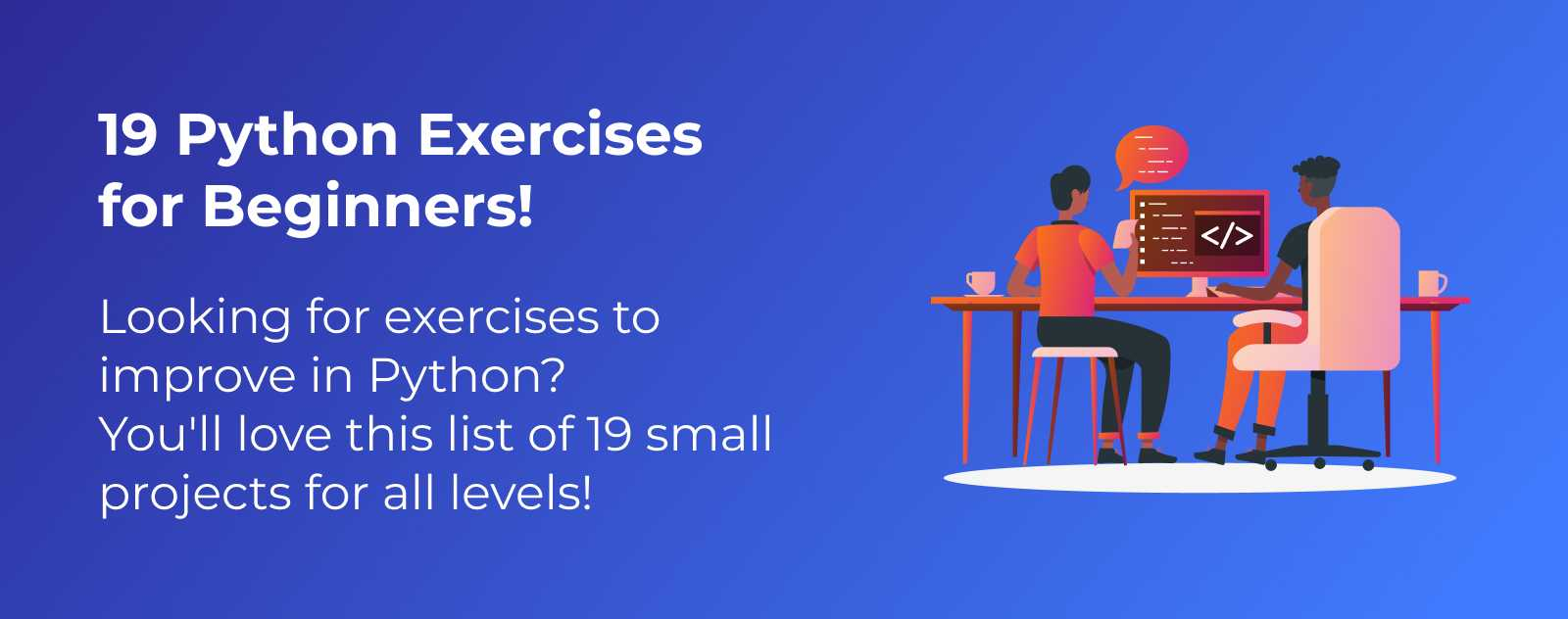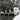# 19 Python Exercises for Beginners!

Article updated on Sunday, November 26, 2023.Looking for exercises to improve in Python? Want to get better at Python but not sure what to do?

The best way to learn coding is through practice!

You’ll discover numerous concepts that will be useful in your daily programming when you create more complex programs.

💡 By the way, if you’re looking for longer projects, then my article 9 Python Project Ideas for Beginners will surely interest you.

Let’s dive right into this list of the best exercises to do to learn Python!

## Exercise 1: Display one or more words with the print function

This first exercise serves as a “Hello world!” Display a string of your choice using the `print()` function in Python.

## Exercise 2: A loop that displays all elements of a list

Continuing with the `print` function but using a loop and a list this time. Loop through a list and display each element of the list.

## Exercise 3: A function that sums a list of numbers

Write a function that adds up each element of a list of numbers and then displays it. Essentially, you’re rewriting the native `sum()` function.

## Exercise 4: Celsius/Fahrenheit Temperature Converter

Write a script that reads a number with the `input()` function and displays its conversion from Fahrenheit to Celsius. To go further, you can propose 2 modes: Celsius to Fahrenheit and Fahrenheit to Celsius. Allow the user to choose a conversion mode with another `input`.

## Exercise 5: Display even numbers between two numbers

Write a script that gives all even numbers between two numbers.

The easiest interpretation of this exercise might be to hardcode a number and then give all even numbers between 0 and that number.

You can go further by using a list or by using the `input` function to make your program interactive.

## Exercise 6: A function that checks if a number is prime

Write an `is_prime()` function that returns `True` or `False` depending on whether the number given to the function is a prime number or not.

## Exercise 7: A loop that displays elements of a dictionary

Create a loop to display all elements of a dictionary. To go further, you can display the key and value of each element of a dictionary independently, formatting them in the way you choose with the `print()` function.

## Exercise 8: Display the Fibonacci sequence up to a given number

Code a program that displays the Fibonacci sequence up to a number. This number can be hardcoded into your code or requested from the user with the `input()` function.

## Exercise 9: Display the vowels in a string

Create a small program that displays the vowels in a string.

## Exercise 10: Display odd numbers in a list

Code a script that takes a list of numbers and displays the odd numbers in that list.

## Exercise 11: Display the total number of characters in a list of words

Program a script that displays the total number of characters in a list of words.

To go further, for example, transform your code into a function that takes lists as parameters, check that they are indeed lists containing words and not other types of variables.

## Exercise 12: Reverse the order of elements in a list

Create a program that changes the order of elements in a list by reversing them.

`[1, 2, 3, 4]` becomes `[4, 3, 2, 1]`.

## Exercise 13: Calculate the factorial of a number

Code a program that gives the factorial of a number.

As a reminder, the factorial is the result of the multiplication of all numbers less than or equal to a given number.

For example, for 6 we have 1, 2, 3, 4, 5, and 6. So to get the factorial of 6 (!6) we multiply 1 x 2 x 3 x 4 x 5 x 6 = 720.

The product of !6 is 720.

## Exercise 14: A function that checks if the word is a palindrome

A palindrome is a word that can be read in both directions, like “Bob” for example.

Write a function that checks if the word is a palindrome or not, returning `True` or `False`.

## Exercise 15: Display a word alternating between uppercase and lowercase

You may know the meme of SpongeBob that aims to mock someone by taking their sentence and alternating the letters between uppercase and lowercase?

Well, today you’re going to code your generator to alternate the letters between uppercase and lowercase because that’s exactly what you’re asked to do in this exercise!

## Exercise 16: Calculate the average of numbers in a list

Create a program that calculates the average of numbers in a list.

## Exercise 17: Display the largest number in a list

Create a program that displays the largest number in a list.

## Exercise 18: Display the longest string in a list

Create a program that displays the longest string in a list of words.

## Exercise 19: Display the numbers in a list that are divisible by 3

Create a program that displays the numbers in a list that are divisible by 3.

## Take Your Python Learning Further!

You now have plenty of exercises to improve in Python.

As I mentioned at the beginning of this article, I wrote an article 9 Python Project Ideas for Beginners for those who want to design longer projects in Python but don’t have too many ideas.Hey, I'm Thomas 👋 Here, you'll find articles about tech, and what I think about the world. I've been coding for 20+ years, built many projects including Startups. Check my About/Start here page to know more :).